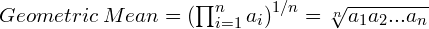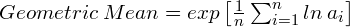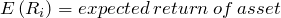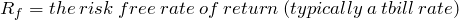## A Close Look at the Math Behind Leveraged ETF Returns

Apr 292011

#### An Application of the Geometric and Arithmetic Means

Over the past several years there has been a proliferation of ETFs for every imaginable purpose.

One particularly interesting type of ETF is the leveraged ETF. These ETFs are designed to provide 2X or 3X the daily return of a target index such as the S&P500, and they do a reasonable job of achieving this goal.

For example, if the S&P500 is up 1% for the day, the 2X S&P500 fund will be up 2% and the 3X fund will be up 3%. If the S&P500 is down 0.5% for the day, then the 2X fund will be down 1% and the 3X fund will be down 1.5%. (Note that for simplicity I’m not considering the fees and implicit borrowing costs associated with leveraged funds in this post).

Based on this description, you might think that if the S&P500 is up 10% for the year, then the 2X S&P500 fund would be up 20% and the 3X S&P500 fund will be up 30%. However, this is not the case. In fact, under some conditions, the 2X and 3X leveraged funds may be up even less than the S&P500 index. How can this be? If the daily leveraged returns are 2X or 3X the returns of the target fund, then why won’t the annual returns be 2X or 3X the annual returns?

To understand why there is discrepancy between daily returns and long-term returns, we need to review the difference between the arithmetic and geometric means.

##### Arithmetic Mean

The arithmetic mean is what most of us think of when we hear the term “average”, and it is defined by this equation.##### Geometric Mean

The geometric mean is the nth root of the product of n numbers, and it is defined by this equation:The geometric mean can also be calculated using logarithms:The geometric mean only applies for positive numbers. So, when using investment returns, the geometric mean is calculated using the decimal multiplier equivalent values. For example, a 6% return is 1.06, and a -4% return is 0.96.

## Is the Market Overvalued?

Apr 252011

Robert Shiller and Jeremy Siegel were on CNBC this past week debating stock market valuation. Shiller makes the case that the market is overvalued based on the historically high PE10 ratio which I wrote about several months ago.

Siegel is more bullish. He argues that valuations are attractive based on the current PE ratio, and he believes the current equity risk premium is quite high. In other words, he believes the expected return on stocks is high relative to the expected return on bonds.

What do you think? Do you agree with Siegel or Shiller?

## Open Yale Courses: Financial Theory

Apr 222011

Earlier this month, Yale University announced announced that an additional ten courses would be available through the “Open Yale Courses” website.

Readers of this site may find the “Financial Theory” course taught by hedge fund manager John Geanakoplos to be especially interesting.  So far, I have only watched the first two lectures, but I’m planning to work through the rest of the course over the next several weeks.

Here is the first lecture:

## Screencast: Fama-French Regression Tutorial Using R

Apr 192011

In this video post, I walk  through a basic demo showing how to run the Fama-French regression using R.

This is my first attempt at doing a screencast, so please let me know if you have any comments or suggestions.

The demo is easiest to follow when viewed in full screen HD.  In lower resolutions, the on-screen commands may be difficult to read.

The slides used in this screencast can be accessed here, and the CSV data file is available here.

The R code used in the demo is shown below:

# Fama-French Regression example in R

# Load CSV file into R

# Extract Fama-French Factors and Fund Returns
rmrf <- ff_data[,2]/100
smb <- ff_data[,3]/100
hml <- ff_data[,4]/100
rf <- ff_data[,5]/100
fund <- ff_data[,6]/100

# Calculate Excess Returns for Target fund
fund.xcess <- fund - rf

# Run Fama-French Regression
ffregression <- lm(fund.xcess ~ rmrf + smb + hml)

# Print summary of regression results
print(summary(ffregression))


## Performance Ratios: The Sharpe Ratio and Beyond

Apr 122011

#### Evaluating Investment Alternatives Using Performance Ratios

In this post, I look at several ratios which are used to evaluate investment performance.

The Sharpe ratio, Treynor ratio, and information ratio are all common ratios for evaluating investment managers and investment portfolios.  Each of these measures can be used ex post to evaluate past performance, or they can be used ex ante to help investors make portfolio decisions based on forecasts for various investment alternatives.

The Sharpe Ratio

The Sharpe ratio is one of the most common metrics for evaluating portfolios.

The Sharpe ratio is calculated by dividing the mean excess return of the portfolio by the standard deviation of the excess return.  In other words, this ratio measures the “reward” we can get for a particular level of variability or  “risk”.

The equation for calculating the Sharpe ratio is:## Jack Bogle – Press on Regardless!

Apr 062011

Jack Bogle was on CNBC earlier this week, and I always enjoy hearing what he has to say.

I agree with Mr. Bogle’s views on market valuation and the outlook for stock returns, but I disagree with him on international diversification.  However, I always remind myself of Bill Bernstein’s well known quote: “When I disagree with Jack Bogle – I am usually wrong.”

Here are a few links to some other interesting Jack Bogle videos  (more interviews with Jack Bogle are available at morningstar.com):

Bogle on Commodity Investing

Bogle: Past is Not Prologue

Bogle on Financial Reform

## Tutorial: The Capital Asset Pricing Model

Apr 032011

Let’s begin with some Q&A:

Question: Why do some financial assets tend to earn higher returns than others?

Answer: Financial theory tells us that, on average, higher returns are earned by financial assets that have higher risk.

Question: Ok, so how do we measure risk?

Answer: One model used for measuring risk is the Capital Asset Pricing Model or CAPM.   In the CAPM, the risk that a security contributes to a diversified portfolio is measured by its beta (or), and the expected excess return of the security is proportional to beta.

The term “excess return” refers to the additional return that the security earns above the risk-free rate.  For U.S. investors, the t-bill rate is typically used as the risk-free rate.

The excess return earned by the market portfolio is also known as the “market risk premium”.  The risk premium for an individual security may be higher or lower than the market risk premium depending on the security’s beta.

If the beta is greater than one (the beta of the market portfolio is one by definition), then the expected return for the security is higher than the market average, and, if the beta is less than one, the expected return is lower.

In the CAPM, the total expected return of a security is given by this equation:Notice that only the excess return varies with beta, and the risk free rate is added to the security’s risk premium to get the total expected return.

The variables in this equation are:The “expected return” is the return that the investors expect to earn on average given the current price of the security.  Of course, the expected return is what should happen on average over the very long term, but in any given period the realized return may be very different than the expected return.

All else equal, the expected return is related to price.  If the price rises, and our expectations of the future cash flows from the security are unchanged, then our expected return falls since we are paying more for the same future future cash flows.  Similarly, if the price falls, and our expectations for future cash flows are unchanged, then the expected return will rise.